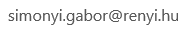﻿ BSM

## Graph Theory

• Instructor: Gabor Simonyi
• Contact:• Prerequisites: Some degree of mathematical maturity is needed for this relatively fast paced course. No prior knowledge of graph theory is required.
• Text:R. Diestel, Graph Theory (available as a digital edition, too) + handouts

Course description: A brief overview of the basic concepts of graph theory will be followed by an in-depth discussion of some classical chapters of graph theory as well as some aspects of a current area: information theoretic graph theory.

Topics:
Basics: Graphs, degrees, paths and cycles, independent sets, cliques, isomorphism, sub- graphs, complement of a graph, trees, Euler tours.
Hamilton cycles: sufficient conditions for Hamiltonicity, theorems of Dirac, Ore, Posa, and Chvatal, the optimality of Chvatal’s theorem.
Matchings: matchings in bipartite graphs, Hall’s theorem and K ?onig’s theorem, Tutte’s theorem, stable matchings, Gale-Shapley theorem.
Colorings: The concept of chromatic number, its relation to the clique number, Mycielsky’s construction, coloring edges, Vizing’s theorem, list coloring, choice number, its relation to the chromatic number, list coloring conjecture, Galvin’s theorem.
Planarity: Euler’s formula, Kuratowski’s theorem, coloring of planar graphs, list coloring of planar graphs.
Basics of extremal graph theory: Tur ´an’s theorem and related results, graph Ramsey theorems.
Perfect graphs: Basic examples, perfectness of comparability graphs, the Perfect Graph Theorem, the Strong Perfect Graph Theorem.
More advanced topics on colorings: Fractional chromatic number, the chromatic number of Kneser graphs and Schrijver graphs.
Capacities of graphs: Products of graphs, Shannon capacity and its bounds, connection to Ramsey numbers, Sperner capacity and other related concepts originated in information theory.